# Two resistors

Two resistors 20 Ω and 60 Ω are connected in series and an external voltage of 400 V is connected to them. What are the electrical voltages on the respective resistors? Please comment!

U1 =  100 V
U2 =  300 V

### Step-by-step explanation: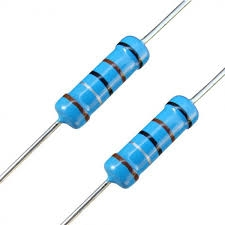Did you find an error or inaccuracy? Feel free to write us. Thank you!Tips to related online calculators
Check out our ratio calculator.

## Related math problems and questions:

• Lowest voltage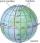Three resistors with resistors R1 = 10 kΩ, R2 = 20 kΩ, R3 = 30 kΩ are connected in series and an external voltage U = 30 V is connected to them. On which resistor is the lowest voltage?
• Resistors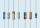Two resistors connected in series give the resulting resistance 245Ω and 60Ω in parallel. Determine the resistance of these resistors.
• Serial connectionIf the voltage on the first resistor is 6 V and the resistance ratio of the first and second resistors is 1: 3, what will be the voltage across the second resistor if they are connected in series?
• Kirchhoff's lawTwo resistors with 100 Ω and 300 Ω resistors are connected in series. A current of 1.8 A passes through the first 100Ω resistor. What current flows through the second resistor?
• Current in the conductorCalculate the current in the conductor (in mA) if it is connected to a 4.5 V voltage source and its resistance is 20 (ohm).
• Circuit voltageCalculate the closed-circuit voltage. Calculate the power on the resistors. R1 = 30 ohms, R2 = 10 ohms. I = 0.1A. U =?. The resistors are connected in series, one after the other.
• Effective and mean voltage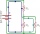A voltage divider consisting of resistors R1 = 103000 Ω and R2 = 197000 Ω is connected to the ideal sine wave voltage source, R2 is connected to a voltmeter which measures the mean voltage and has an internal resistance R3 = 200300 Ω, the measured value i
• Mains voltageHow much electric current flows through the appliance with a resistance of 40kΩ, which is connected to the mains voltage (230 V)?
• The bulbsThe bulbs are connected serially two bulbs and one resistor. Each bulb has a resistivity of 100 ohms and a resistor of 60 ohms. The voltage source in the circuit is 24 volts. Do you know what voltage to measure on individual appliances?
• Filament of bulbThe filament of bulb has a 1 ohm resistivity and is connected to a voltage 220 V. How much electric charge will pass through the fiber when the electric current passes for 10 seconds?
• Closed circuitIn a closed circuit, there is a voltage source with U1 = 12 V and with an internal resistance R1 = 0.2 Ω. The external resistance is R2 = 19.8 Ω. Determine the electric current and terminal voltage.
• Resistor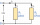Resistor 1 with a resistance of 100 Ohms and resistor 2 with a resistance of 400 Ohms are connected side by side in the circuit. There is a voltage of 80V between the resistor terminals. a) Draw a circuit diagram and write the entered quantities in it b)
• TransformerSolve the textbook problems - transformer: a) N1 = 40, N2 = 80, U2 = 80 V, U1 =? b) N1 = 400, U1 = 200 V, U2 = 50 V, N2 =?
• R1+R2 in parallelThe resistance ratio of the first and second resistors is 3: 1 and the current flowing through the first resistor is 300 mA. What current flows through the second resistor (resistors connected in parallel)?
• Coils of transformerWhat voltage is generated on the secondary coil of the transformer, which has 1500 turns, if the primary coil is connected to a voltage of 120 V and has 500 turns? What is the transformation ratio and what is the transformation about?
• The bulbWhat is the resistance of a filament lamp when it is connected to a 9V battery and has a current of 120 mA?
• Coils of transformerThe primary coil of the transformer has 1100 turns and is connected to a voltage of 220V. How many turns does the secondary coil have when the voltage on it is 55 V? Find the transformation ratio and decide what kind of transformation.